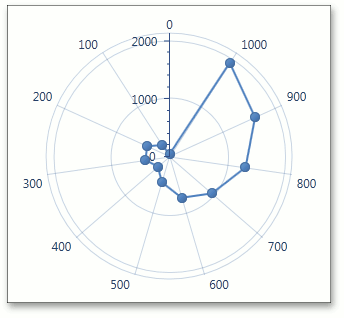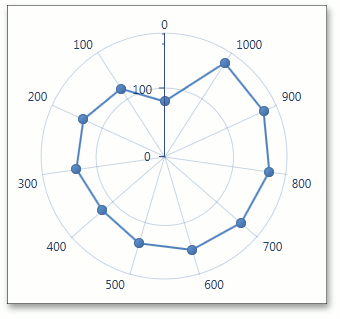20.2 (EAP/Beta)
20.1
19.2
19.1
18.2
18.1
17.2

# CircularAxisY2D.Logarithmic Property

Gets or sets whether the circular Y-axis should display its numerical values using a logarithmic scale.

Namespace: DevExpress.Xpf.Charts

Assembly: DevExpress.Xpf.Charts.v20.1.dll

## Declaration

``public bool Logarithmic { get; set; }``
``Public Property Logarithmic As Boolean``

## Property Value

Type Description
Boolean

true to display the logarithmic scale; otherwise, false.

## Remarks

If a chart's series has its Series.ValueScaleType property set to ScaleType.Numerical, you can use the Logarithmic property to specify whether a logarithmic scale should be used to display axis values. When the Logarithmic property is set to true, you can also define a logarithmic base value via the CircularAxisY2D.LogarithmicBase property.

The following table illustrates the Logarithmic property in action.

Logarithmic = false

Logarithmic = true

LogarithmicBase = 10